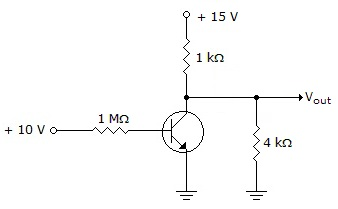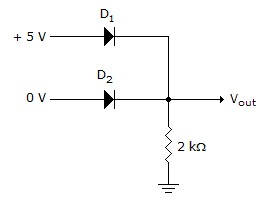# Digital Electronics - Signals and Switches

### Exercise :: Signals and Switches - General Questions

21.

TTL logic chips must have connections to Vcc and ground, even if all inputs and outputs are properly used and tied to valid signals.

 A. True B. False

Answer: Option A

Explanation:

No answer description available for this question. Let us discuss.

22.

What is the output voltage Vout for the given circuit if the input voltage changes to 0 V?A. +12 V B. +15 V C. +3 V D. 0 V

Answer: Option A

Explanation:

No answer description available for this question. Let us discuss.

23.

If the input voltage on D1 in the given figure is changed to 0 V, what is the output voltage Vout?A. –5 V B. 0 V C. +5 V D. +4.3 V

Answer: Option B

Explanation:

No answer description available for this question. Let us discuss.

24.

An indication of cutoff in an NPN bipolar transistor is that the:

 A. collector current is maximum B. collector-to-emitter voltage equals zero C. base-to-emitter voltage equals 0.7 V D. collector to emitter appears to be open

Answer: Option D

Explanation:

No answer description available for this question. Let us discuss.

25.

When an IC has two rows of parallel connecting pins, the device is referred to as a:

 A. QFP B. DIP C. TSSOP D. CMOS

Answer: Option B

Explanation:

No answer description available for this question. Let us discuss.

#### Current Affairs 2021

Interview Questions and Answers